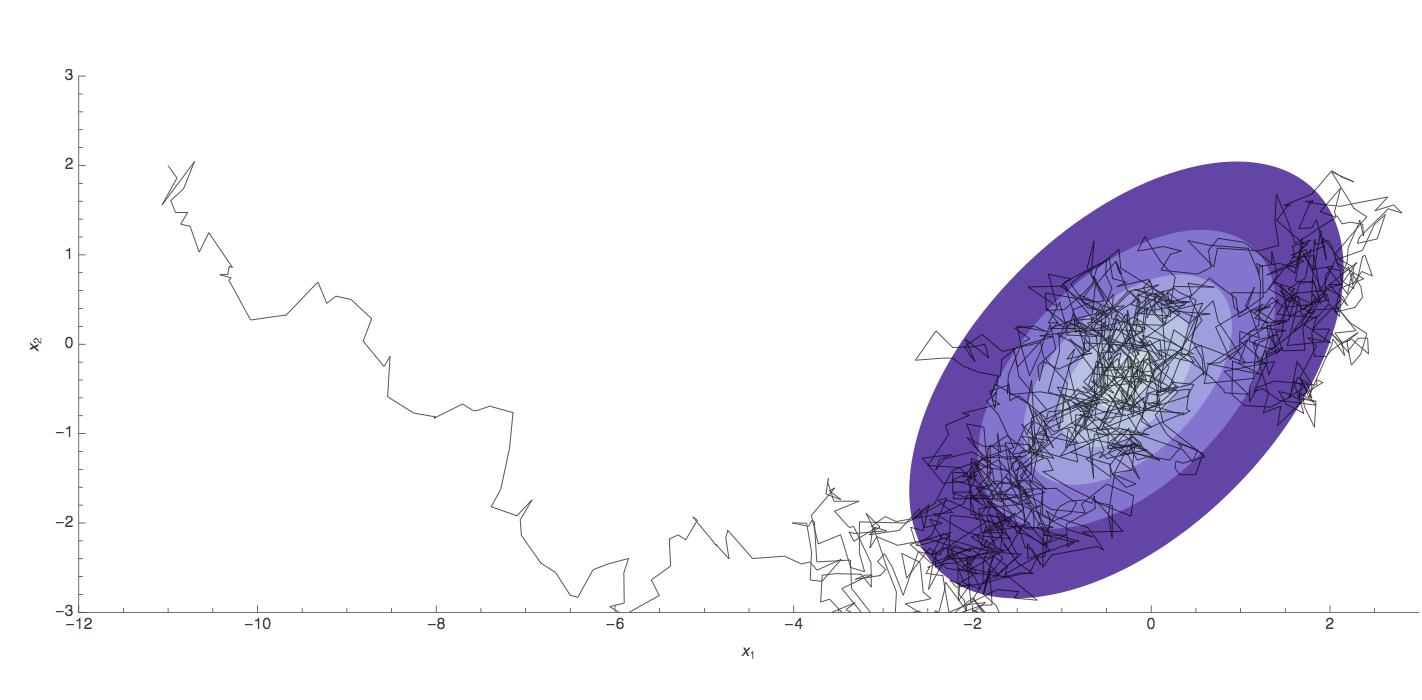## Phylogenetics

### Phylogeny describes evolutionary relationships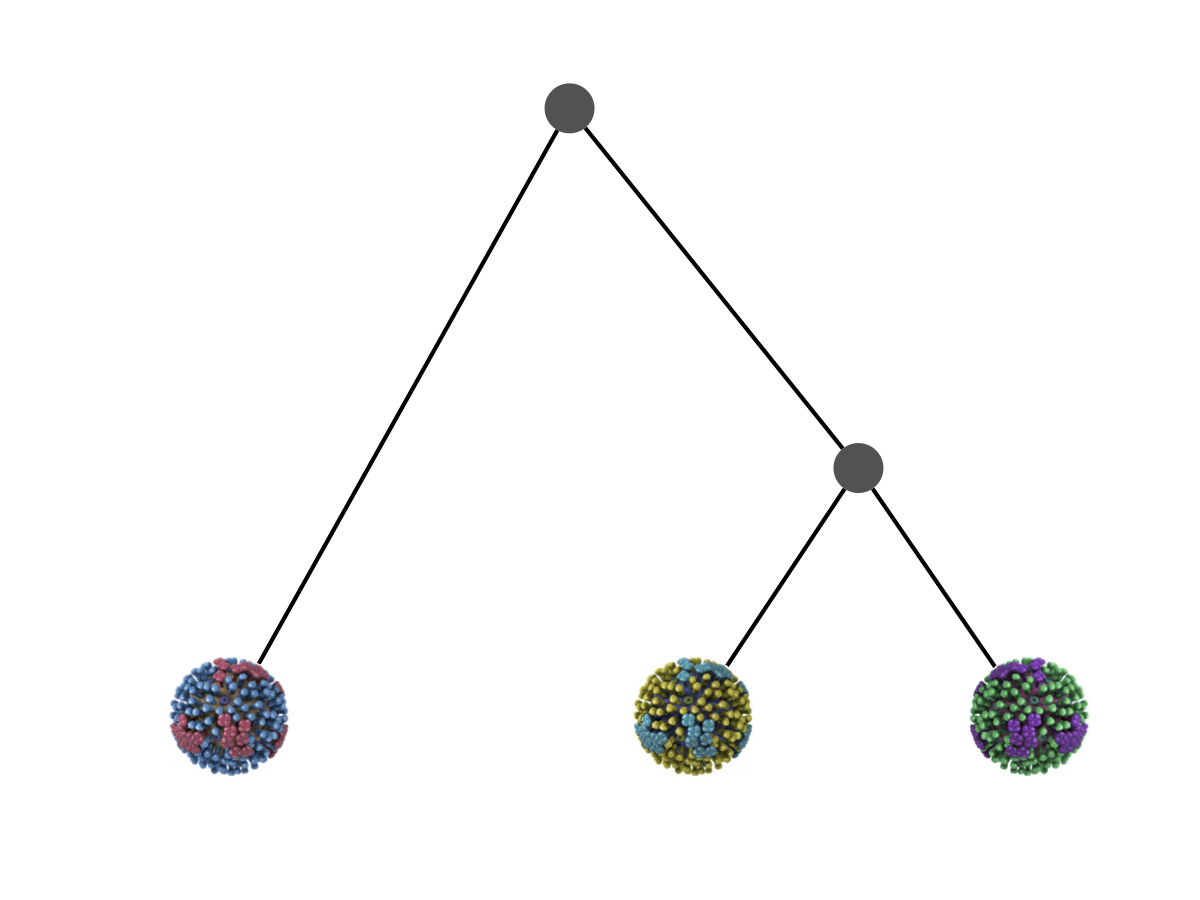### Phylogeny is usually a hypothesis based on characteristics of sampled taxa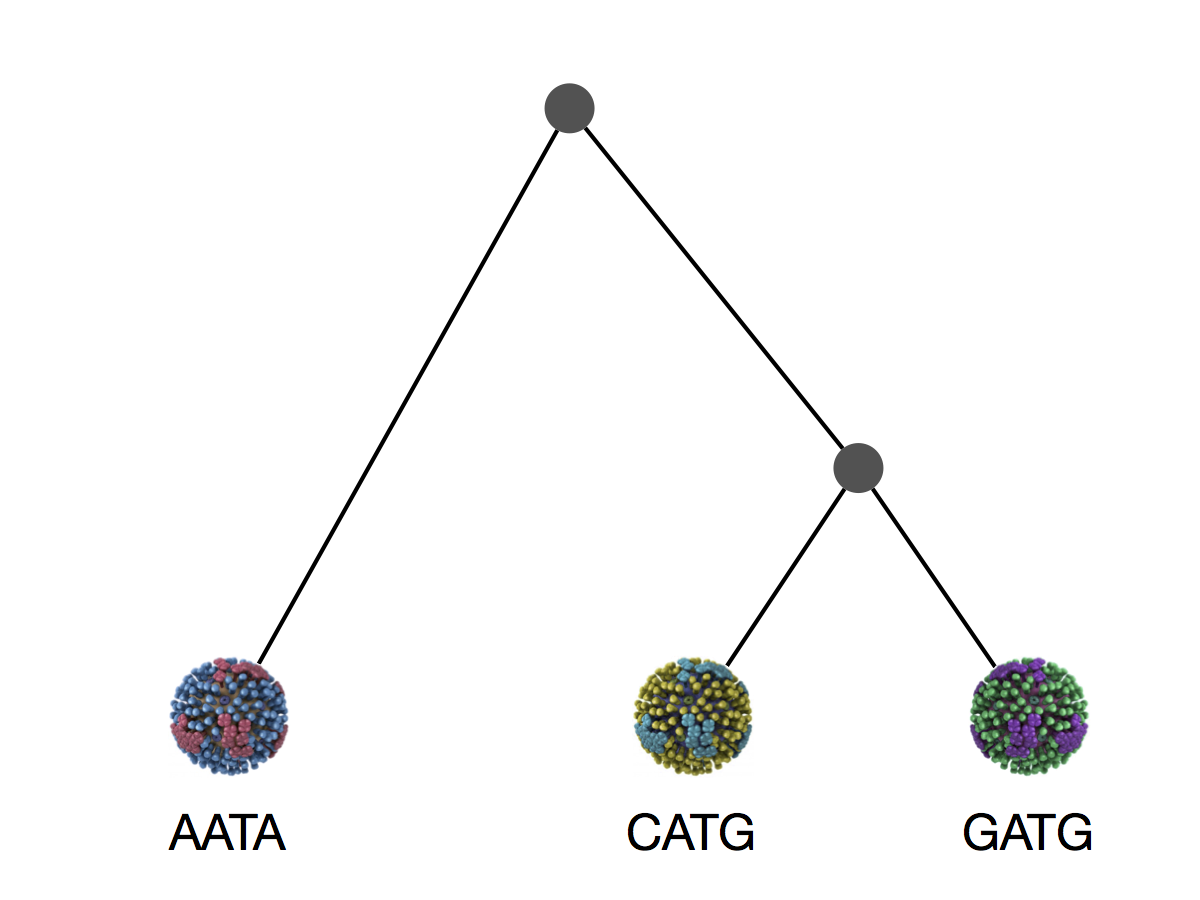### Phylogeny implies a series of mutational events leading to observed tip states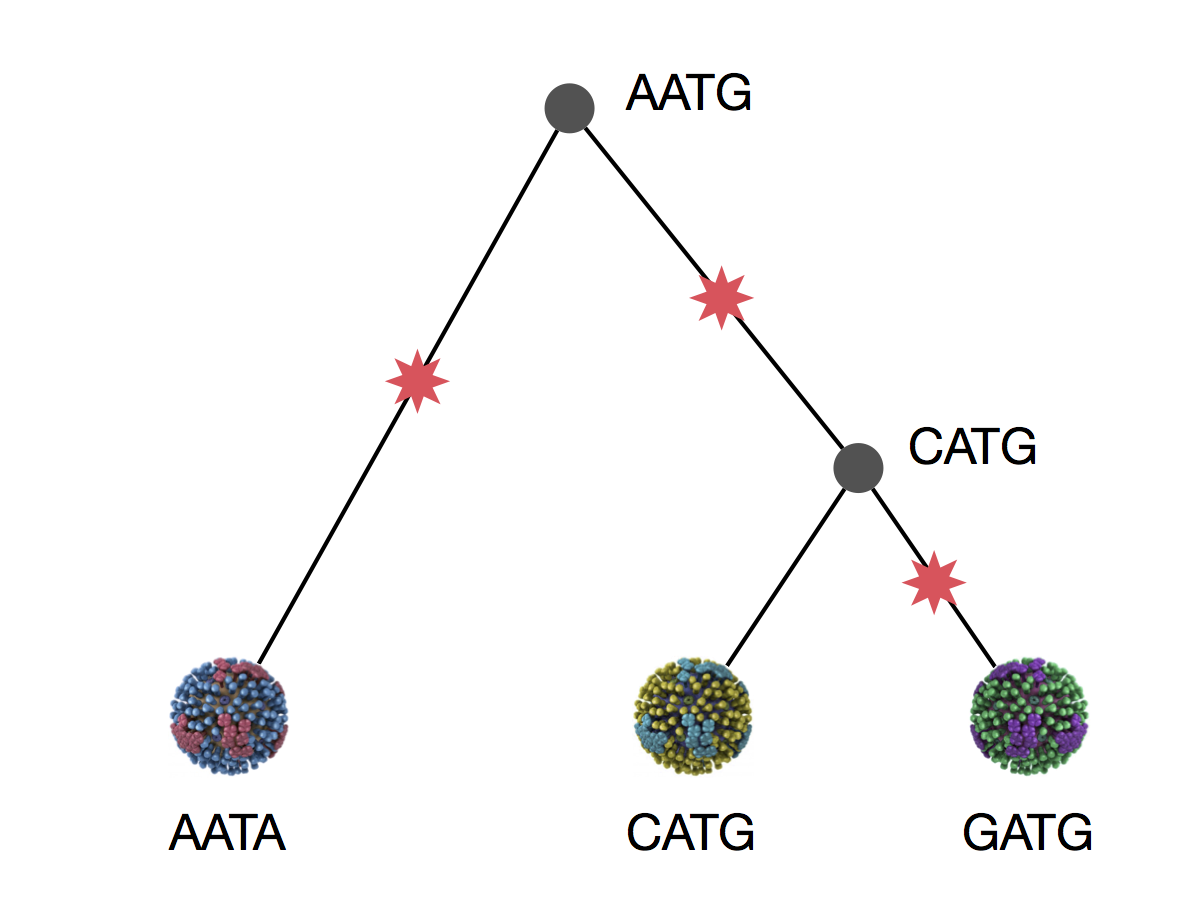### Parsimony is based on Occam's razor

Among competing hypotheses that predict equally well, the one with the fewest assumptions should be selected.

### Parsimony suggests this topology requires 3 mutations at minimum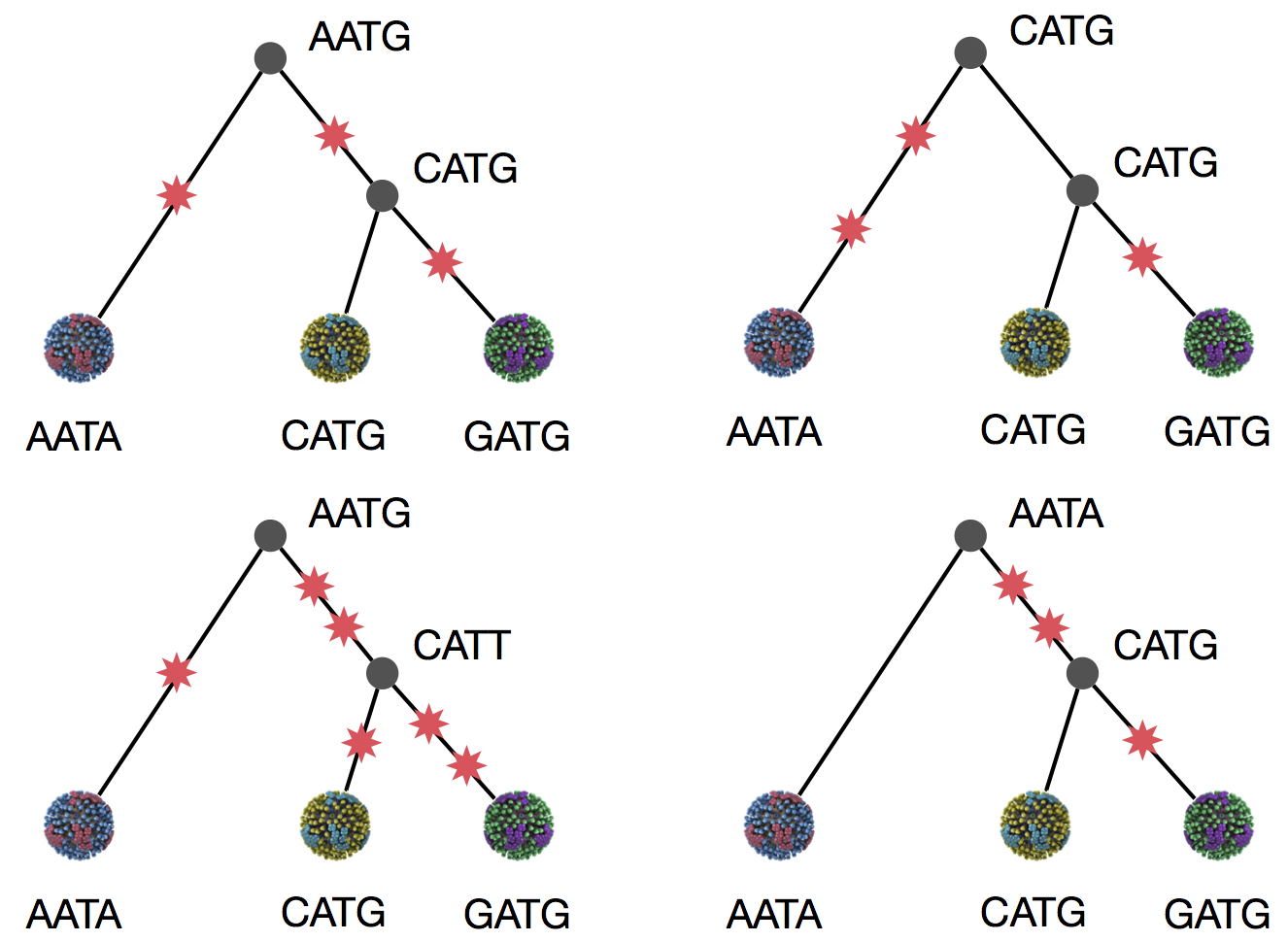### Parsimony suggests both topologies equally tenable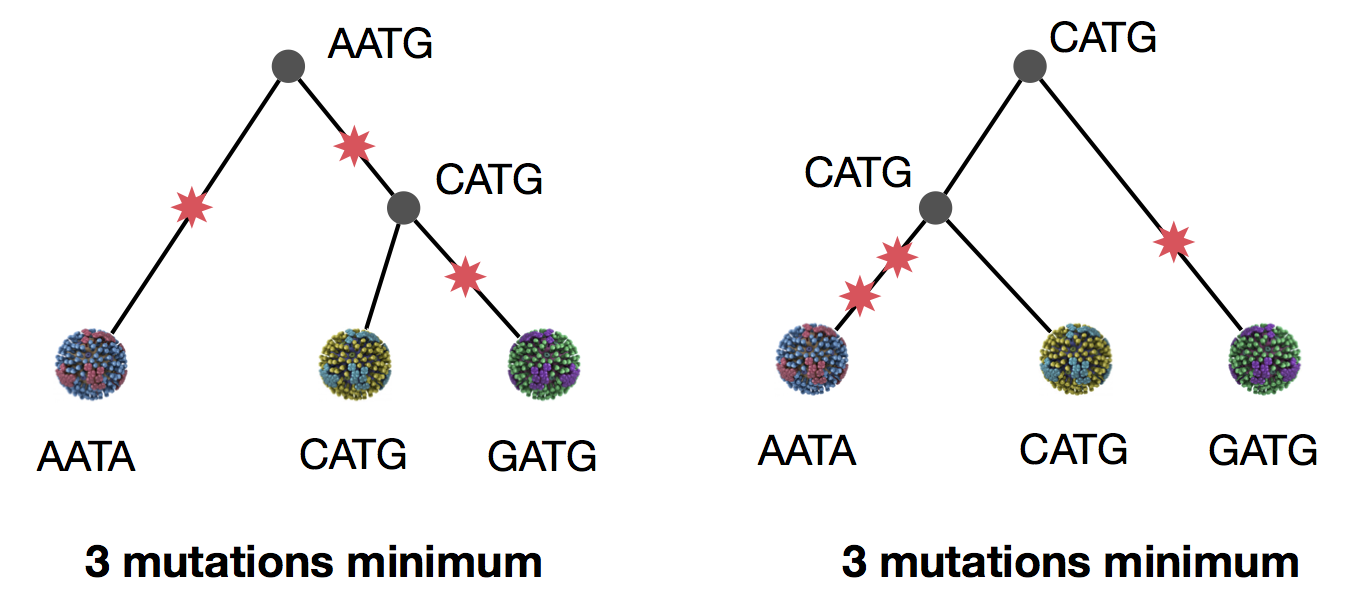### Exercise: which topology is more likely under parsimony?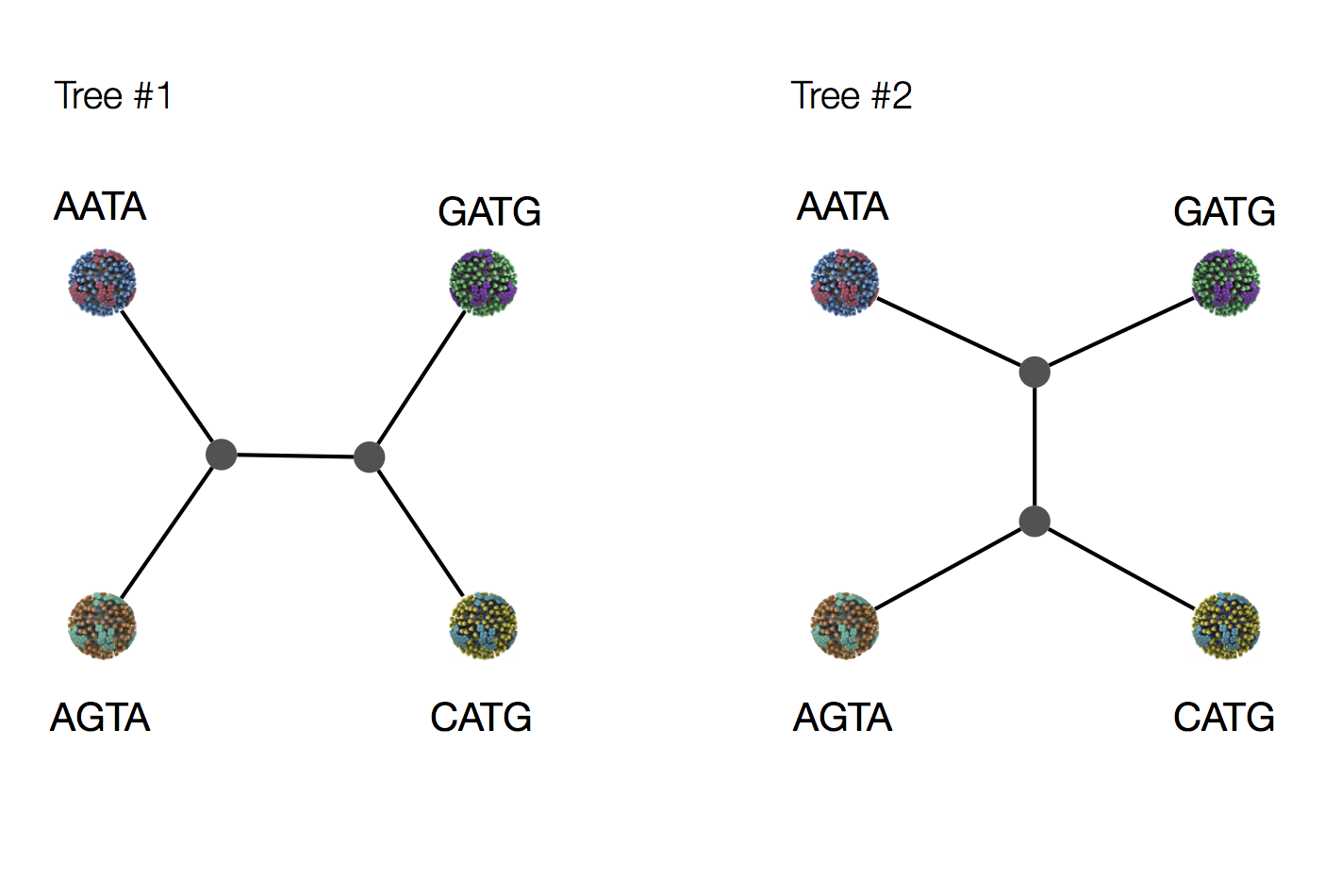### Exercise: which topology is more likely under parsimony?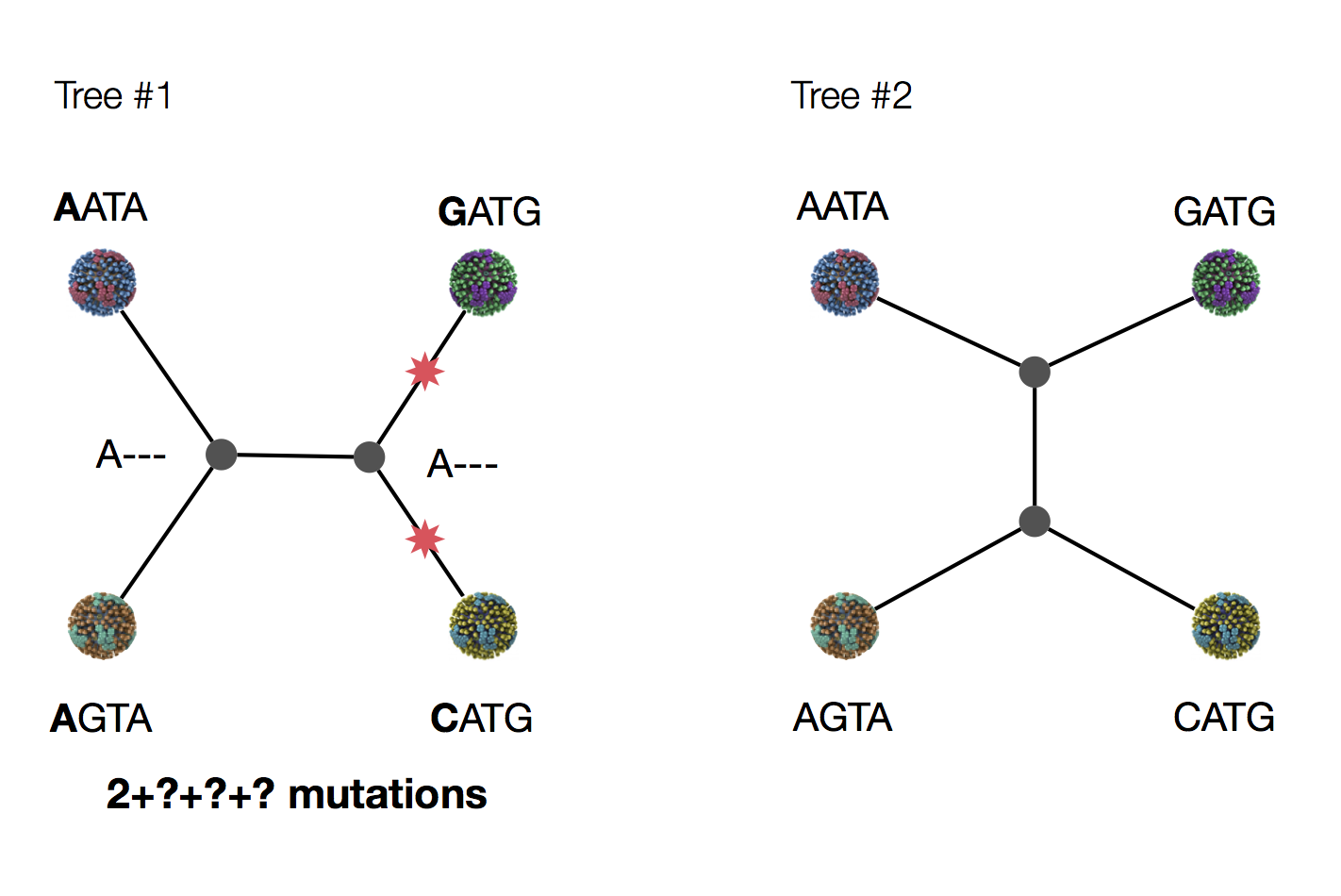### Exercise: which topology is more likely under parsimony?### Exercise: which topology is more likely under parsimony?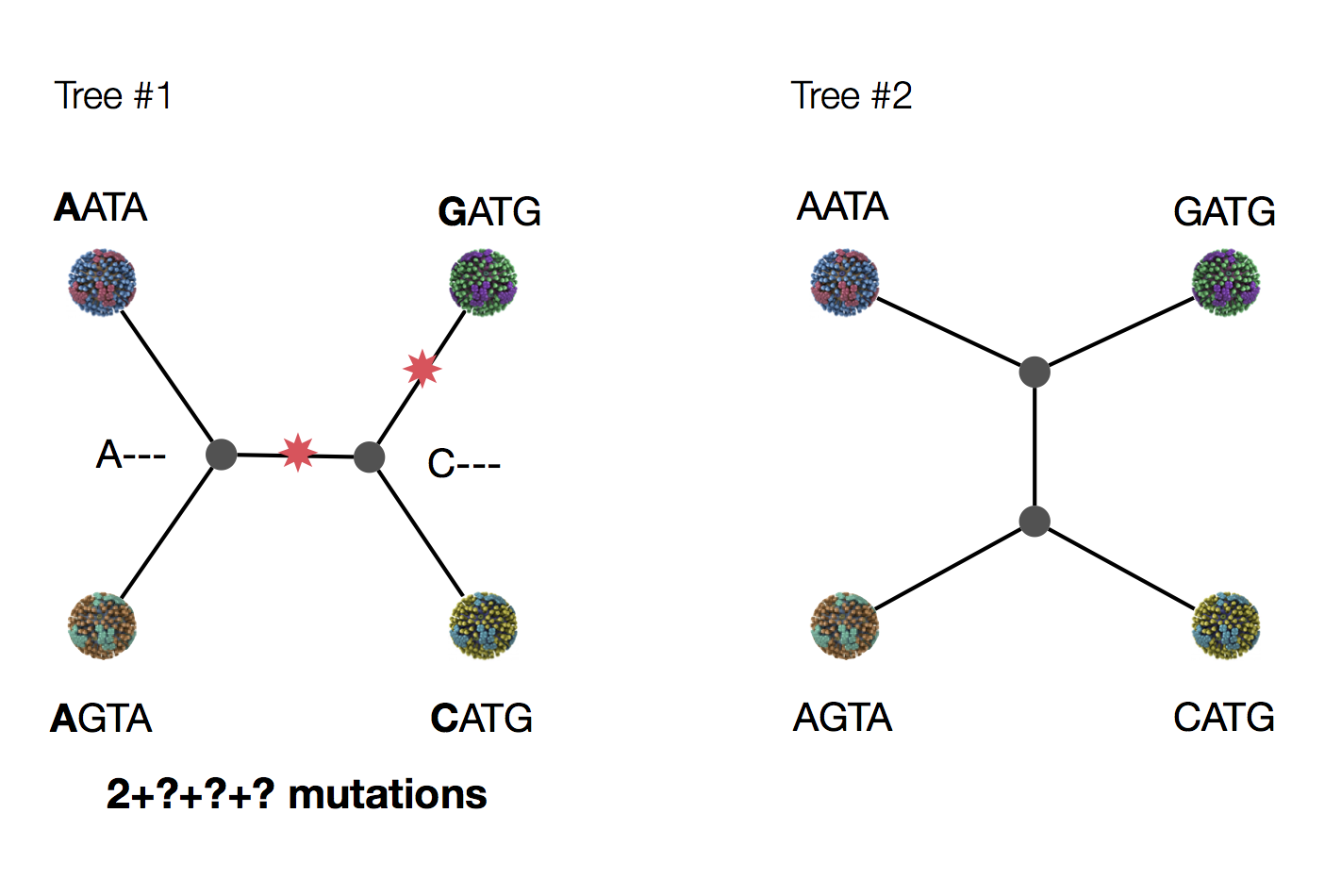### Exercise: which topology is more likely under parsimony?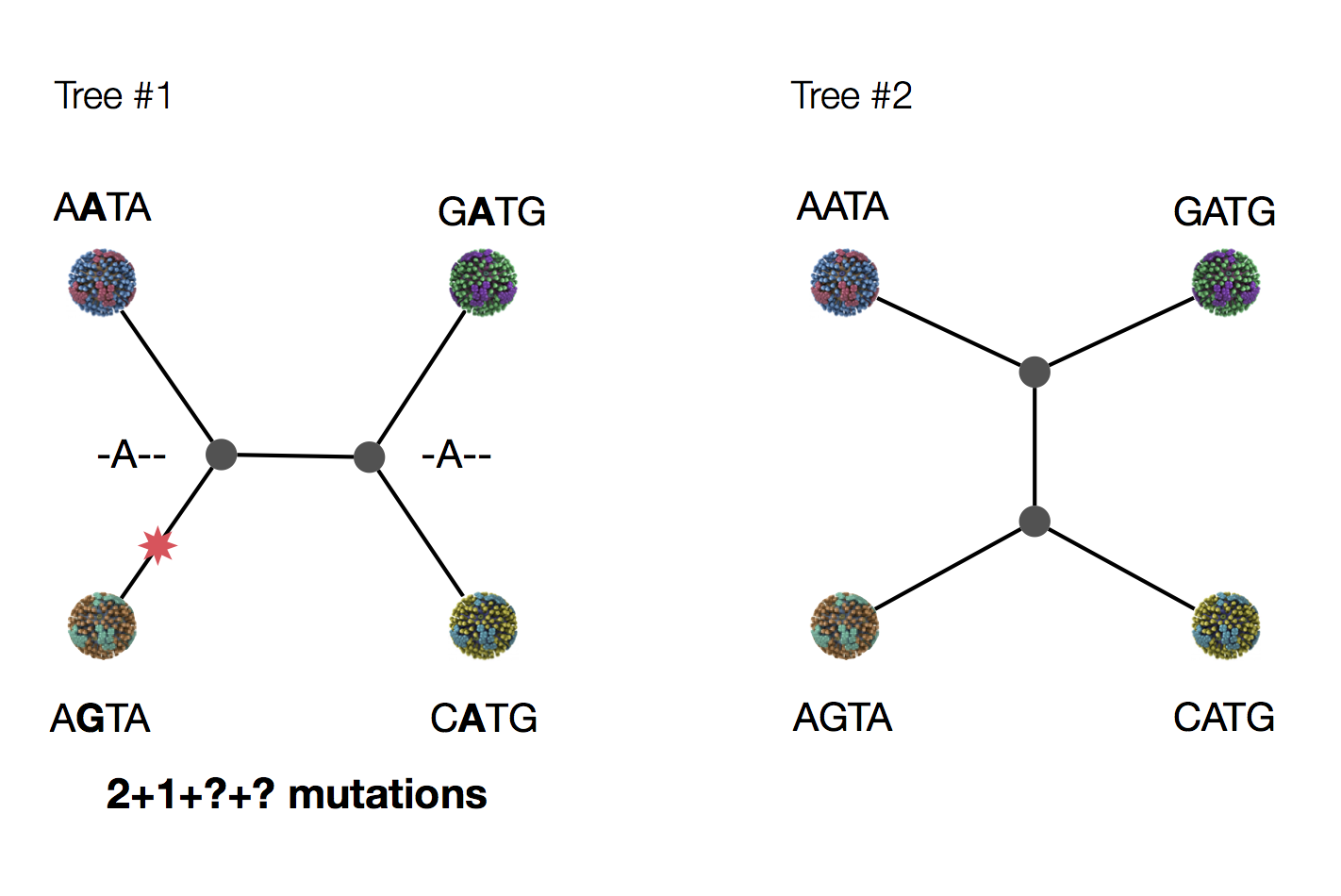### Exercise: which topology is more likely under parsimony?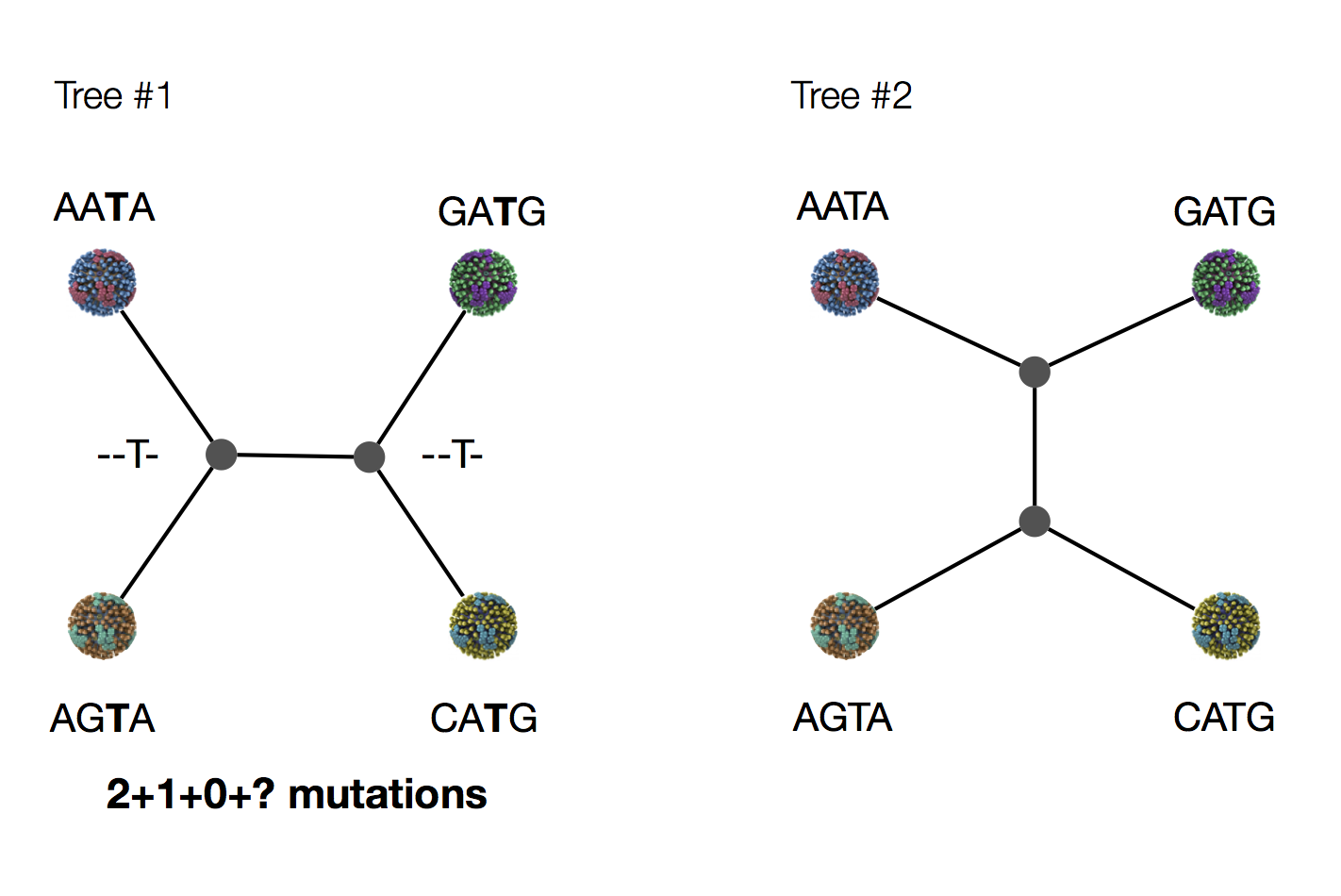### Exercise: which topology is more likely under parsimony?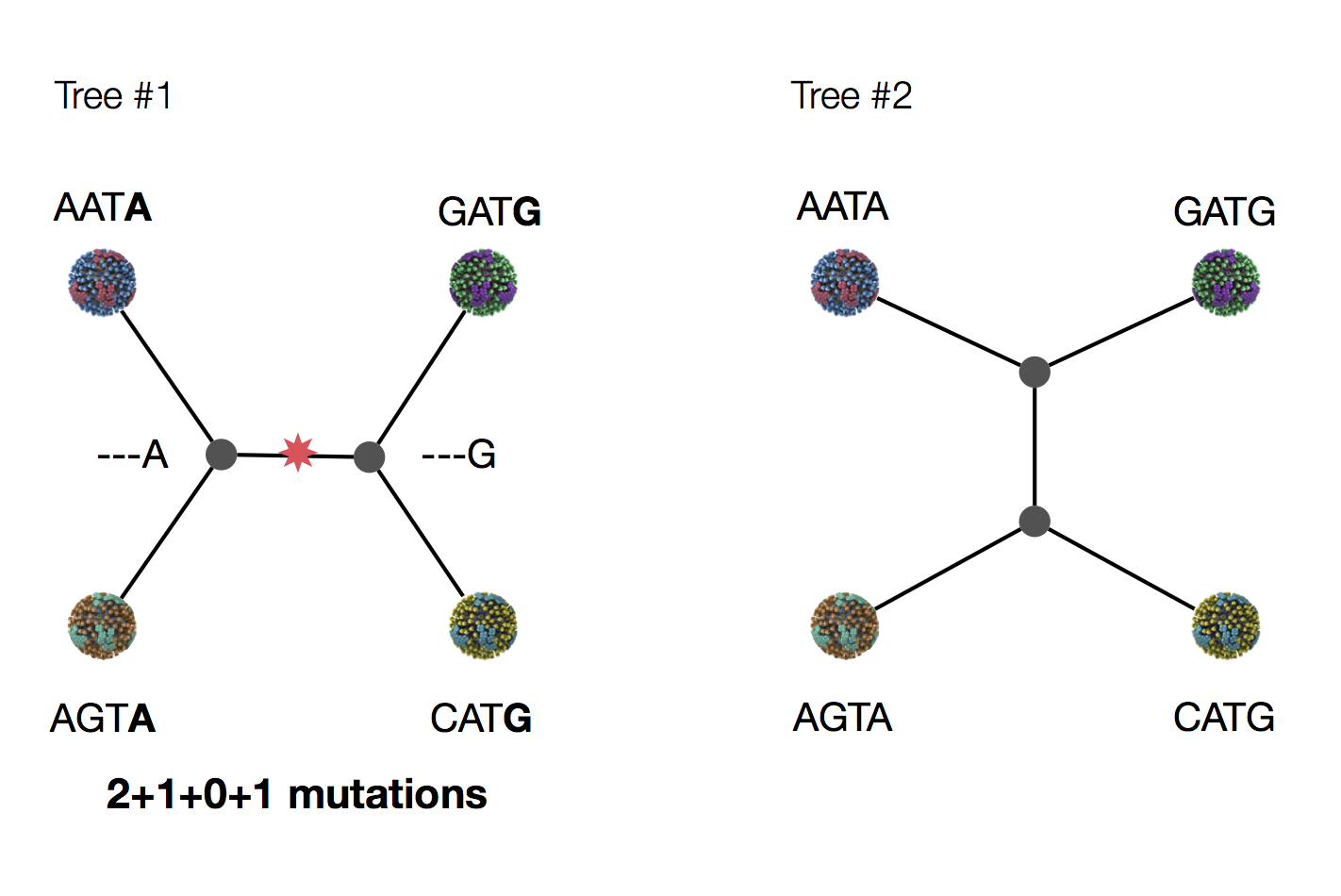### Exercise: which topology is more likely under parsimony?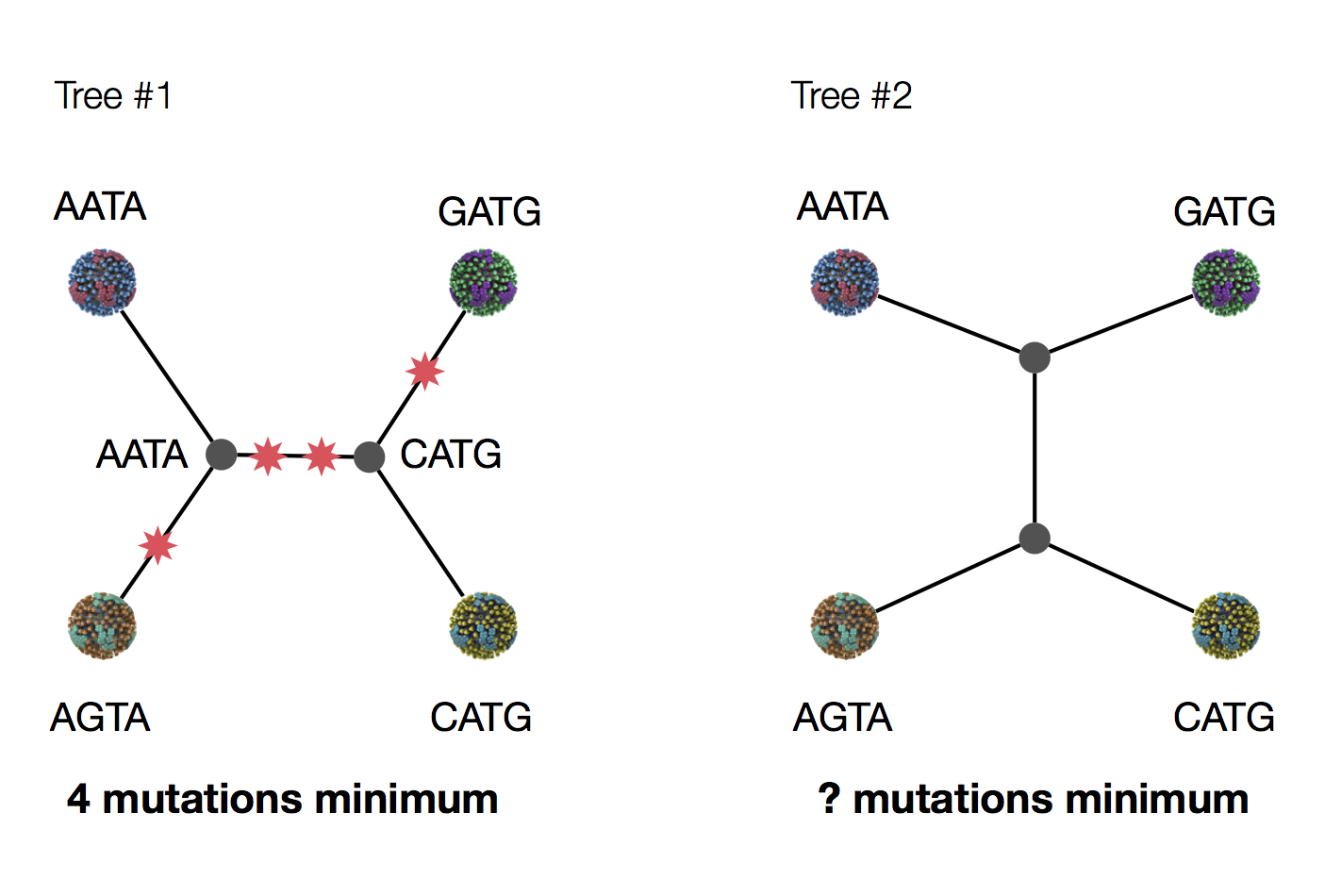## Inferring phylogenies with likelihood

### Maximum likelihood (ML) inference

In ML, you have some set of data $D$ and a model for generating this data. This model has parameters $\theta$. The probability of observing data is $\mathrm{Pr}(D \, | \, \theta)$. The best parameter point estimate $\hat{\theta}$ is simply the value that maximizes $\mathrm{Pr}(D \, | \, \theta)$.

### Maximum likelihood (ML) inference

For example, if we have data $D$ from a Bernoulli observation model representing $k$ successes in $n$ trials, then the probability of observing $k$ and $n$ given coin flip probability parameter $p$ is simply $$\mathrm{Pr}(k,n \, | \, p) = p^k \, (1-p)^{n-k}.$$

### Maximum likelihood (ML) inference

For the Bernoulli model $\mathrm{Pr}(k,n \, | \, p) = p^k \, (1-p)^{n-k}$, we have $\hat{p} = \cfrac{k}{n}$. For example, with $k=8$ and $n=10$, $\hat{p}=0.8$ and the likelihood curve follows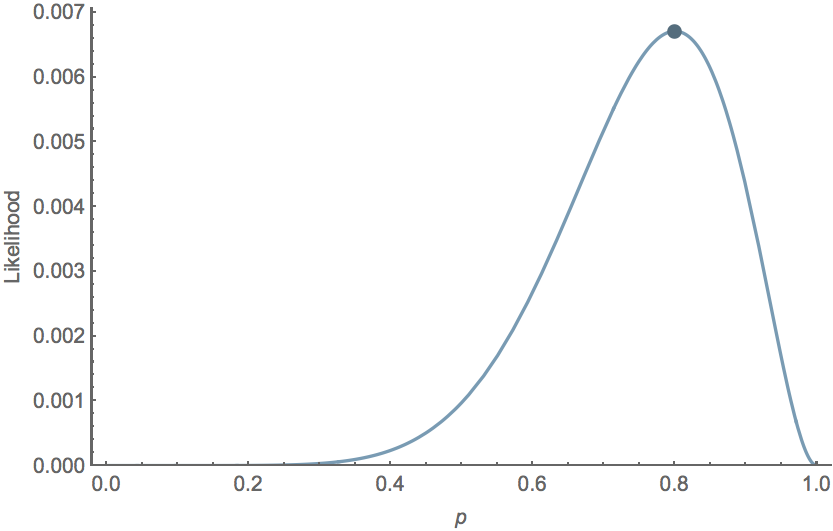### Data likelihood

In phylogenetics, $D$ are the observed tip sequences and $\theta$ is the phylogenetic tree including topology and branch lengths.

### Calculating the likelihood of a single topology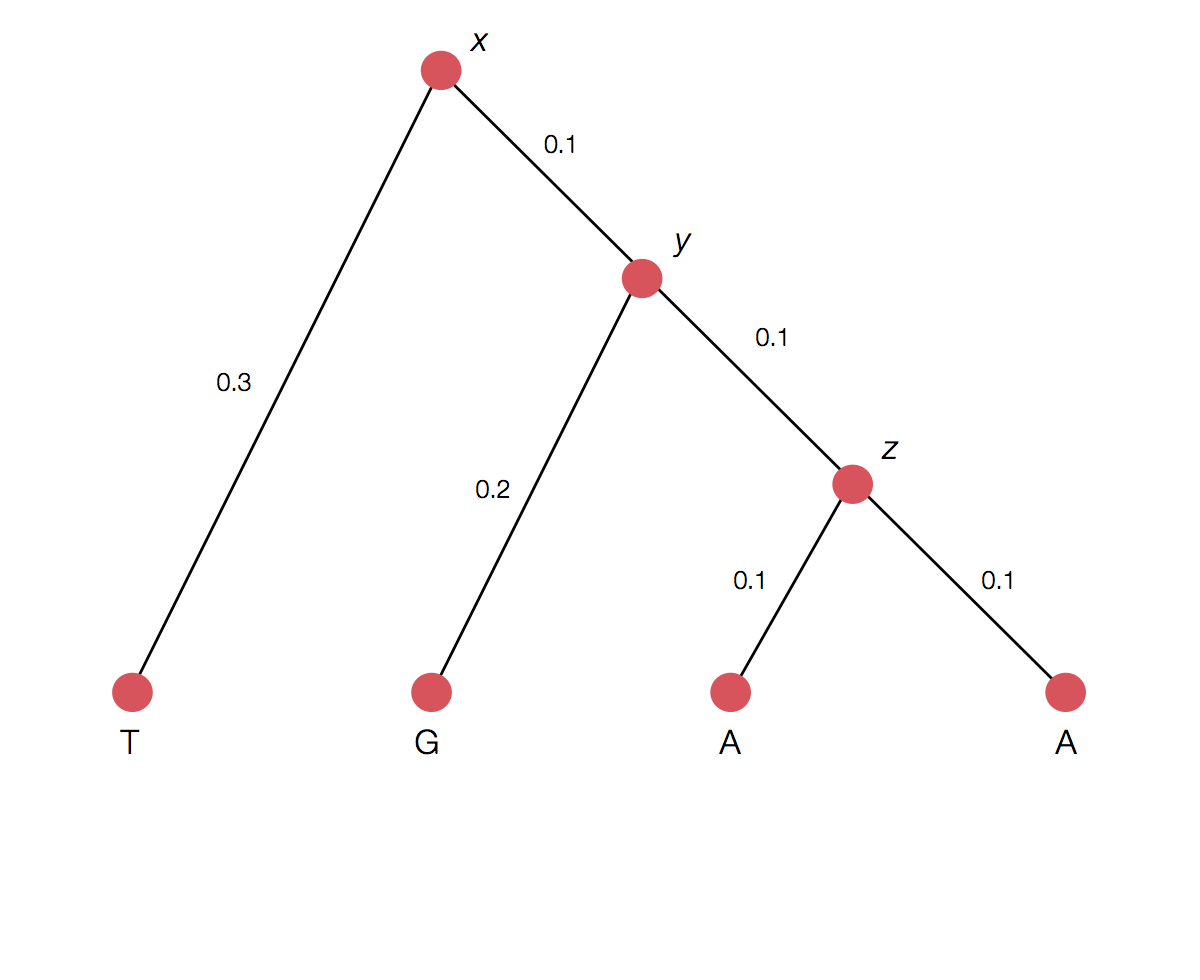### Transition probability for each branch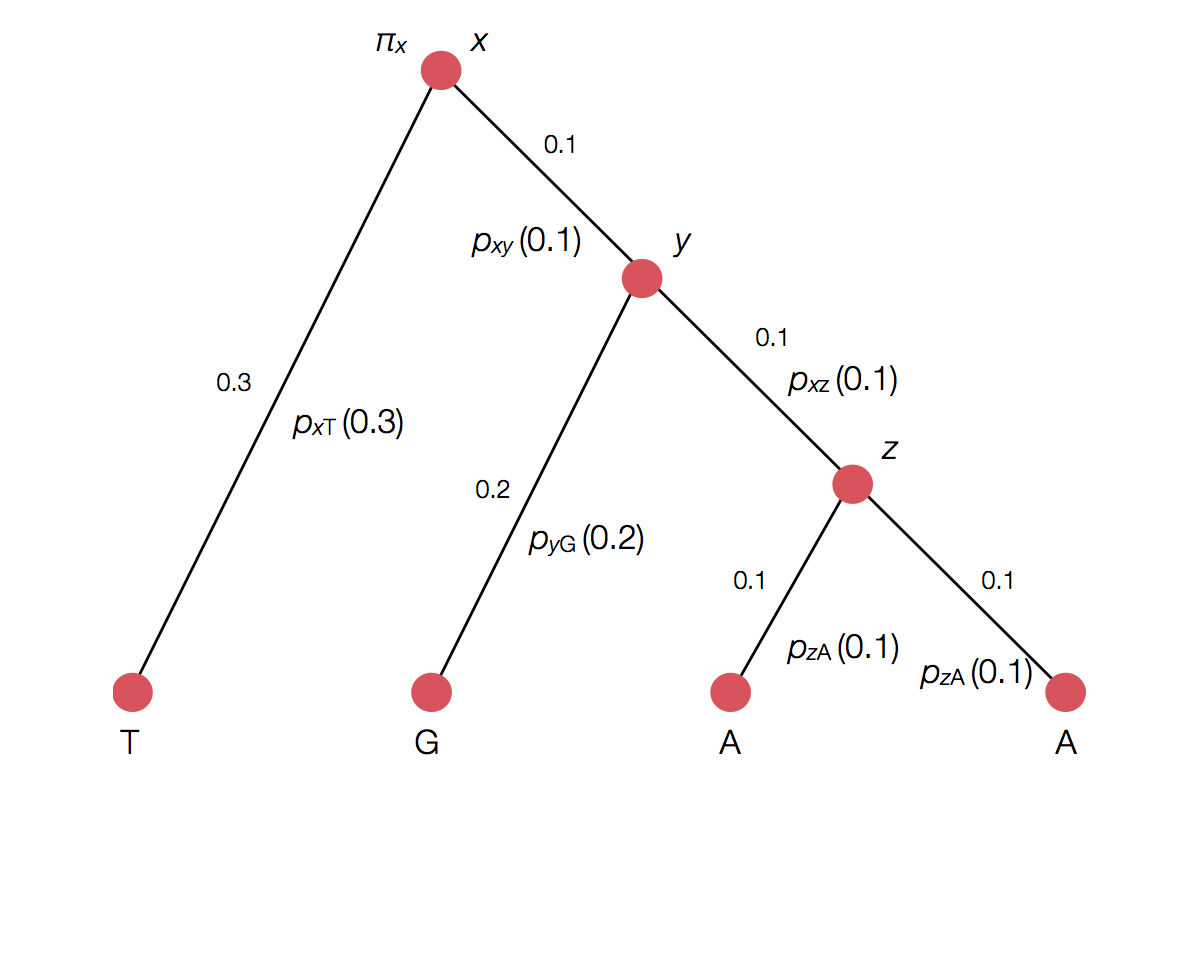### Rather than enumerating all possible internal states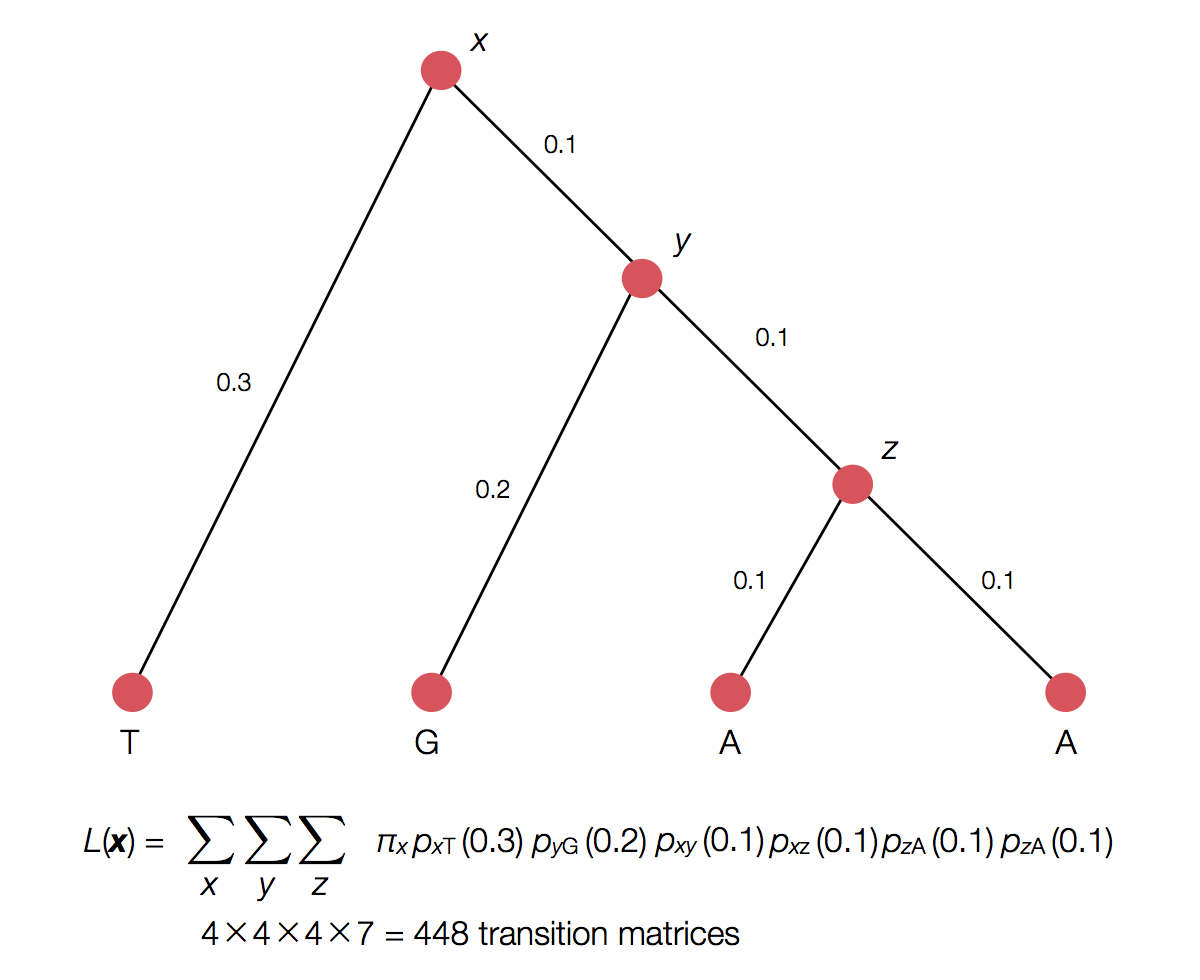### Can use Felstenstein's pruning algorithm to speed up calculation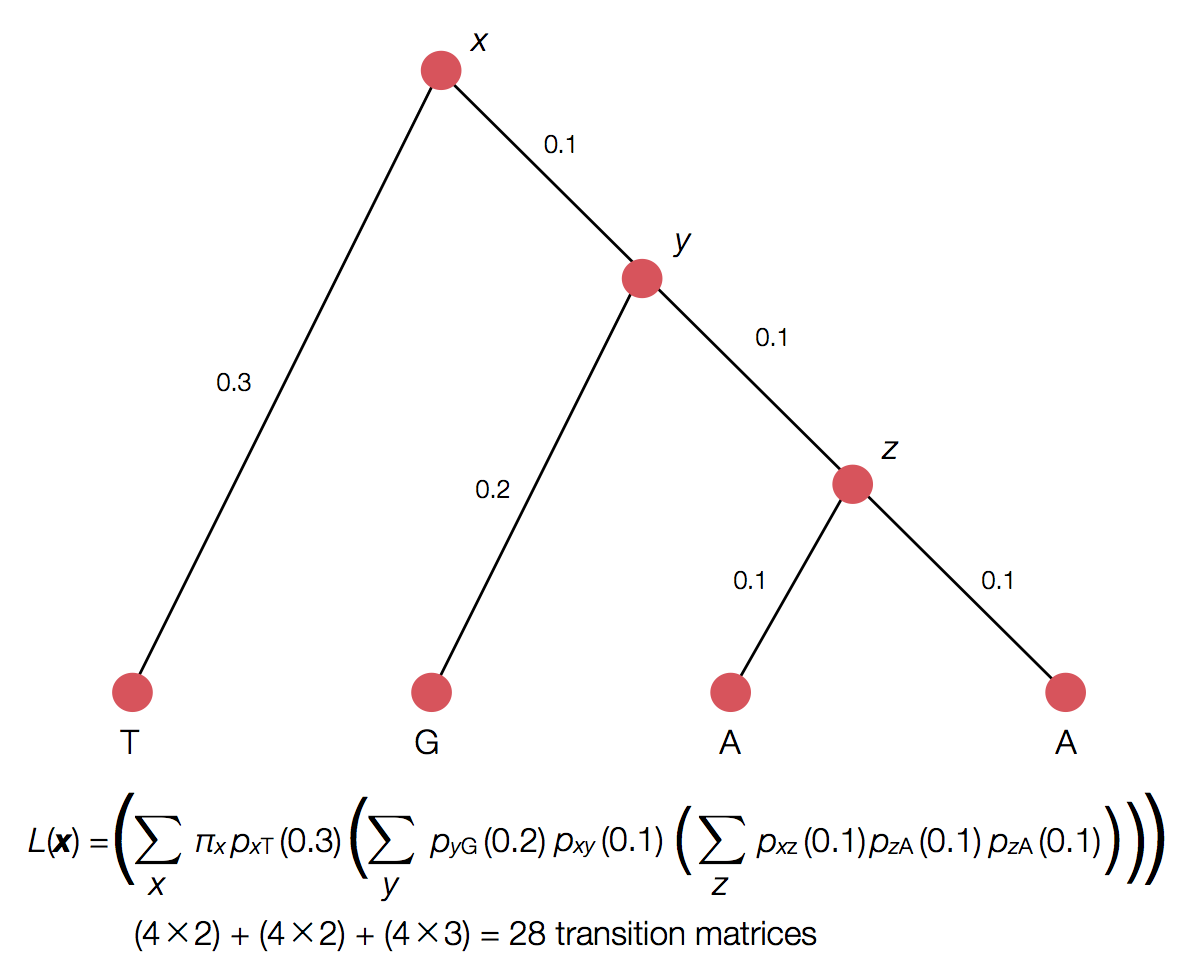### ML inference

Inference becomes a search for the tree that maximizes the likelihood of observing tip sequences. Lots of computation goes into this.

## Bayesian phylogenetic inference

### Bayesian inference

Generally, it's difficult to make probability statements using frequentist statistics. You cannot directly say that model 1 is twice as likely as model 2. People misuse p values in this sort of fashion all the time.

### Bayes' rule

Bayes' rule forms the basis of Bayesian inference, it states: $$\mathrm{Pr}(A \, | \, B) = \cfrac{ \mathrm{Pr}(B \, | \, A) \, \mathrm{Pr}(A) }{ \mathrm{Pr}(B) }$$

### Bayes' rule exercise

For example, let's say we have an Ebola test that is 99% sensitive and 99% specific (meaning if someone has Ebola it will report true 99% of the time and if someone doesn't have Ebola it will report false 99% of the time). Let's further say that 0.1% of the population has Ebola. If we select a random individual and observe a positive test result, what is the probability that they actually have Ebola?

### Bayes' rule exercise

If we select a random individual and observe a positive test result, what is the probability that they actually have Ebola? I.e. $\mathrm{Pr}(\mathrm{P} \, | \, \mathrm{E}) = 0.99$, $\mathrm{Pr}(\mathrm{N} \, | \, \mathrm{E}) = 0.01$,
$\mathrm{Pr}(\mathrm{P} \, | \, \mathrm{NE}) = 0.01$, $\mathrm{Pr}(\mathrm{N} \, | \, \mathrm{NE}) = 0.99$
and $\mathrm{Pr}(\mathrm{E}) = 0.001$.
In this case, what is $\mathrm{Pr}(\mathrm{E} \, | \, \mathrm{P})$?

### Bayesian inference

Bayesian inference applies Bayes' rule in a likelihood context, so that $$\mathrm{Pr}(\theta \, | \, D) = \cfrac{ \mathrm{Pr}(D \, | \, \theta) \, \mathrm{Pr}(\theta) }{ \mathrm{Pr}(D) },$$ where $D$ is data and $\theta$ are parameters. $\mathrm{Pr}(D)$ is constant with respect to $\theta$, so that $\mathrm{Pr}(\theta \, | \, D) \propto \mathrm{Pr}(D \, | \, \theta) \, \mathrm{Pr}(\theta)$. This relationship is often referred to as $\mathrm{posterior} \propto \mathrm{likelihood} \times \mathrm{prior}$.

### Bayesian inference for Bernoulli model

Following our previous Bernoulli example, we've observed $k$ successes in $n$ trials, and so the likelihood $\mathrm{Pr}(k,n \, | \, p) = p^k \, (1-p)^{n-k}$. We'll assume a flat prior $\mathrm{Pr}(p) = 1$. In this case, the marginal likelihood follows $$\mathrm{Pr}(k,n) = \int_0^1 \mathrm{Pr}(k,n \, | \, p) \, \mathrm{Pr}(p) \, dp = \cfrac{k! \, (n-k)!}{(n+1)!}.$$ And the full posterior follows $$\mathrm{Pr}(p \, | \, k,n) = \cfrac{(n+1)! \, p^k \, (1-p)^{n-k}}{k! \, (n-k)!}.$$

### Probability statements

If $k=8$ and $n=10$, the mean posterior $\mathrm{E}[p] = 0.75$, while the 95% credible interval extends from $0.482$ to $0.896$, and the posterior distribution follows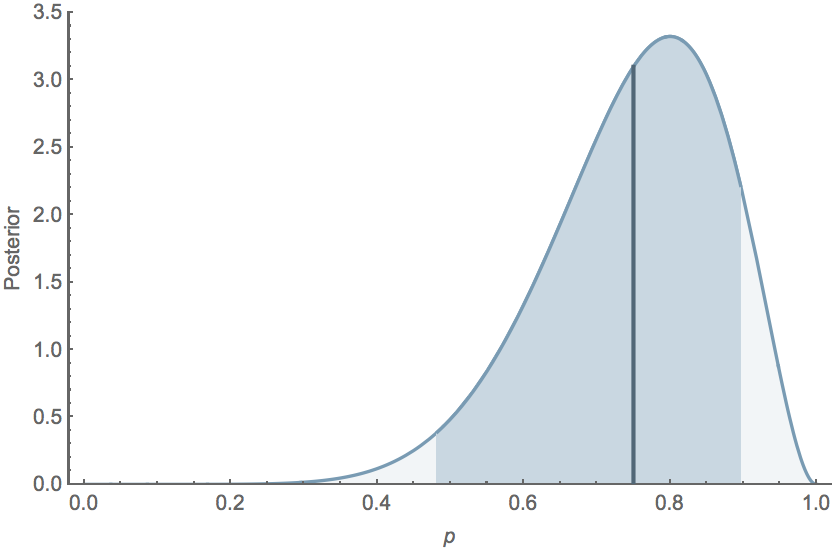### Bayesian phylogenetic inference

Here, we are interested in the posterior distribution $\mathrm{Pr}(\tau, \mu \, | \, D)$, where $D$ represents sequence data, $\tau$ represents the tree topology and $\mu$ represents mutational parameters (like transition vs tranversion rate). In this case, $$\mathrm{Pr}(\tau, \mu \, | \, D) \propto \mathrm{Pr}(D \, | \, \tau, \mu) \, \mathrm{Pr}(\tau) \, \mathrm{Pr}(\mu).$$

### Bayesian coalescent inference

In the case of the coalescent model, we are interested in coalescent rate parameter like $\lambda$. Here, we use $\lambda$ to give the likelihood of observing a particular tree topology $\mathrm{Pr}(\tau \, | \, \lambda)$. This probability is the likelihood of observing the coalescent intervals seen in the tree.

### Bayesian coalescent inference

Thus, the full model becomes $$\mathrm{Pr}(\tau, \mu, \lambda \, | \, D) \propto \mathrm{Pr}(D \, | \, \tau, \mu) \, \mathrm{Pr}(\tau \, | \, \lambda) \, \mathrm{Pr}(\lambda) \, \mathrm{Pr}(\mu).$$ Bayesian approaches work well to build these sorts of nested models.

## BEAST: Bayesian Evolutionary Analysis by Sampling Trees

### Markov chain Monte Carlo (MCMC)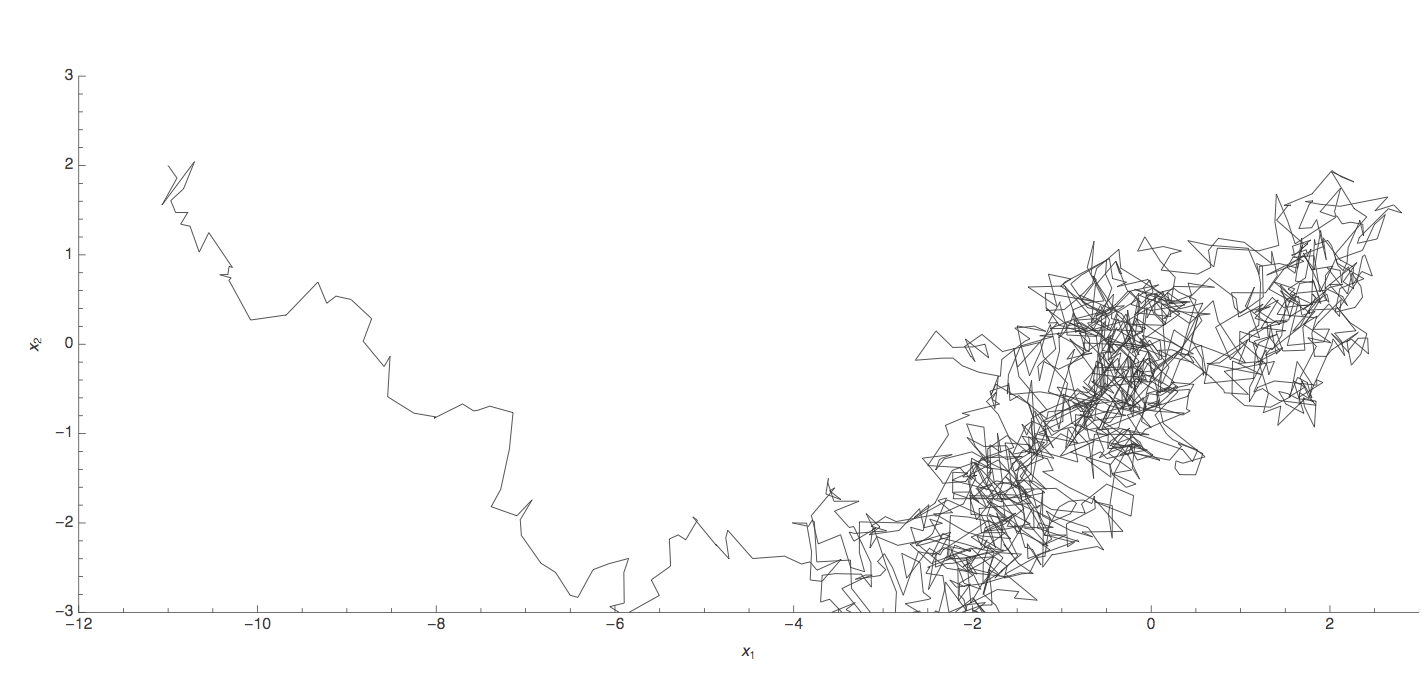### Markov chain Monte Carlo (MCMC)